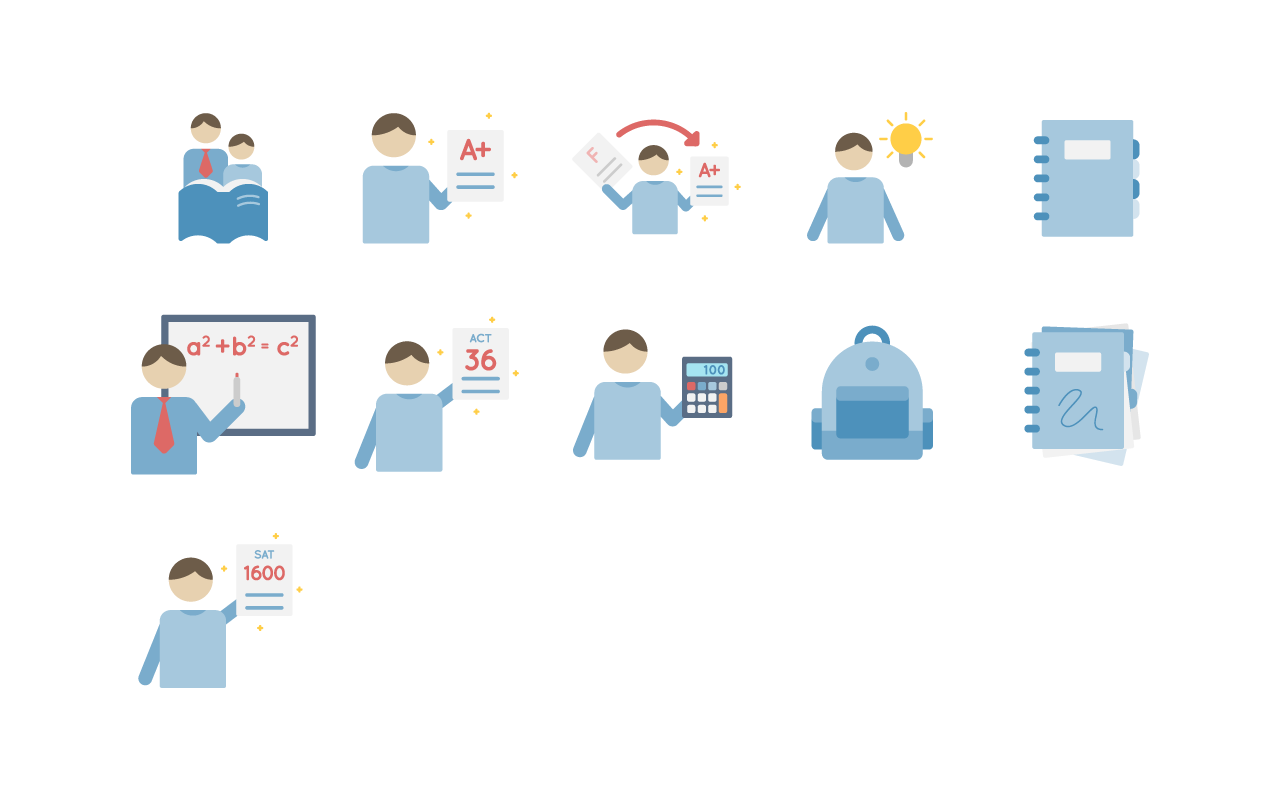If you're in need of premium tutor icons, you came to the right place. You have permission to use any of the icons from the tutor icon set above as long as you include a link to the author's website (bocatutor.me). Here are all of the files in the tutor icon set available in zip format. Inside you'll find svg and png files. Download Tutor Icon Set

We hope you'll find these icons useful for your latest project. The tutor icon set is perfect for a math tutor, science tutor, reading tutor, SAT tutor, ACT tutor, and tutoring centers. If you'd like to request that an icon be added to this set, please contact us with ideas you have.

This icon is perfect not only if you're a reading tutor, but also if you tutor math, science, or any other subject that requires a textbook. The icon shows a tutor and a student reading a book together. The tutor is helping the student read and understand the material being read.# A Test

This icon shows a student holding a test paper with an A+ on it. It doesn't have to be a test. It can also be a paper, homework, or anything else that's graded by a teacher or professor. Presumably the tutor helped the student achieve the A+ on this quiz, exam, test, paper, or homework assignment. The grade can be for almost any class such as any math (pre-algebra, algebra 1, algebra 2, geometry, college algebra, trigonometry, statistics, pre-calculus, calculus, and beyond). It can also represent a test or lab assignment for a science class such as (biology, anatomy, physics, and chemistry).# F to A Test

In case you don't like the icon above, but need to convey grade improvement this icon might be useful. This icon demonstrates that the student is improving from F (failing) to A+ (excelling). The math tutor helped the student improve. Of course it doesn't need to be a math tutor. It can be any kind of tutor. There's also an arrow going from the F to the A+, making it clear that the old grade is an F and the new grade is an A+.# Perfect 36 ACT

Some tutors prefer preparing students for standardized tests such as the ACT or the SAT. It's impossible to find good standardized test icons, which is why we're including this in the tutor icon set. In case you don't know, the ACT is a standardized test with a maximum score of 36. The ACT has 4 main sections (English, Reading, Math, and Science). The 5th section, Writing, is optional. This icon works very well if you're an ACT tutor. We might make icons for each of these sections in the future, if there's enough interest.# Perfect 1600 SAT

If you tutor for the ACT, then you probably tutor for the other big standardized test, the SAT. Instead of a maximum score of 36, the perfect score for the new SAT is 1600. This test has 3 main sections (Reading, Math, Writing & Language). There's also an optional Essay. If you need icons for these separate sections, let us know.# Lightbulb

When a tutor explains a difficult concept really well, a lightbulb usually goes off. This icon shows an student with a lightbulb going off right next to his head. The student has an epiphany thanks to the tutor. The tutor might be a math tutor who just showed the student how to solve a difficult integral. He also might be a chemistry tutor who just showed the student how to balance a difficult chemical equation. There are so many possibilities where this icon can be used.# Calculator

Unlike the above icons, this one is a little less flexible. You can really only use this if you're a math tutor, chemistry tutor, biology tutor, or physics tutor. Not many other high school classes require a calculator. The icon shows a student with a calculator in his hand. The calculator shows the number 100. It can be interpreted in a couple of ways. Either the student just solved a difficult calculator problem, or he's asking the tutor to help him use the calculator properly. If you need the student to hold a graphing calculator, let us know, and we'll make a new icon.# Math Problem

Just like the last icon, this one can pretty much only be used if you're a math tutor. It shows a math tutor in a red tie explaining the Pythagorean theorem on a whiteboard. The tutor is writing on the whiteboard using an Expo dry-erase marker. If you need a different problem written on this white board, we are considering making several different versions of this icon. Let us know what you'd like to see in place of the Pythagorean theorem. Once we make some more versions, it can be used by pre-algebra, algebra 1, algebra 2, geometry, college algebra, trigonometry, statistics, pre-calculus, and calculus tutors. We might even make versions that would work well for biology, chemistry, physics, and computer tutors.# Clean Backpack

Some tutors specialize in study skills and organization, and this icon shows an organized and clean backpack. If you're one of these tutors, you'll find this useful since it shows organization.# Messy Binder

Some students perform poorly in school because they aren't organized and can't plan ahead properly. If you look at their binders, you'll find a total mess. Just like the previous icon, this one is meant for tutors who specialize in study skills and organization.# Clean Binder

This serves a continuation to the messy binder icon. Here's what the student's binder should look like after the tutor shows the student how to organize a binder. You can use this in combination with the messy binder, showing a before and after.